# mathematics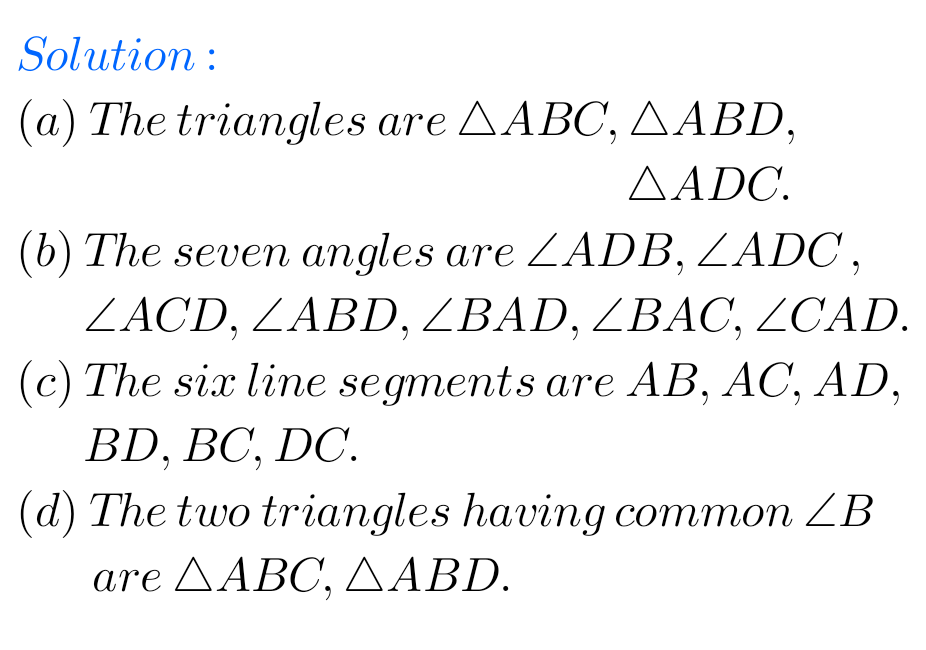## Basic Geometrical Ideas Solutions Class 6 Ncert Maths exercise 4.4

Maths Ncert Basic Geometrical Ideas Exercise 4.4 Solutions Ncert textbook Mathematics Class 6 Chapter 4 Basic Geometrical Ideas exercise 4.4 solutions are given. Study the lesson Basic Geometrical Ideas in the texbook. Observe the problems and solutions given in the text book. Observe the given below problems and solutions and try them in your own …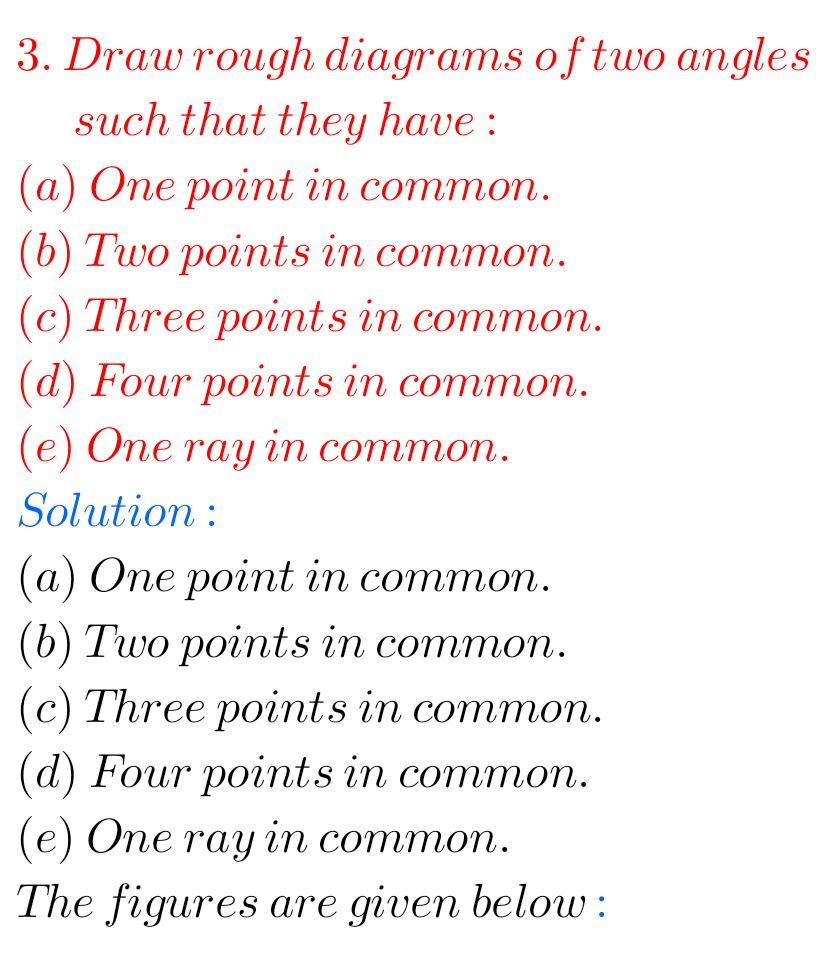## Basic Geometrical Ideas Solutions class 6 exercise 4.3 Ncert Maths

Basic Geometrical Ideas Solutions exercise 4.3 Class 6 Ncert Maths Ncert textbook Mathematics Class 6 Chapter 4 Basic Geometrical Ideas exercise 4.3 solutions are given. Study the lesson Basic Geometrical Ideas in the texbook. Observe the problems and solutions given in the text book. Observe the given below problems and solutions and try them in …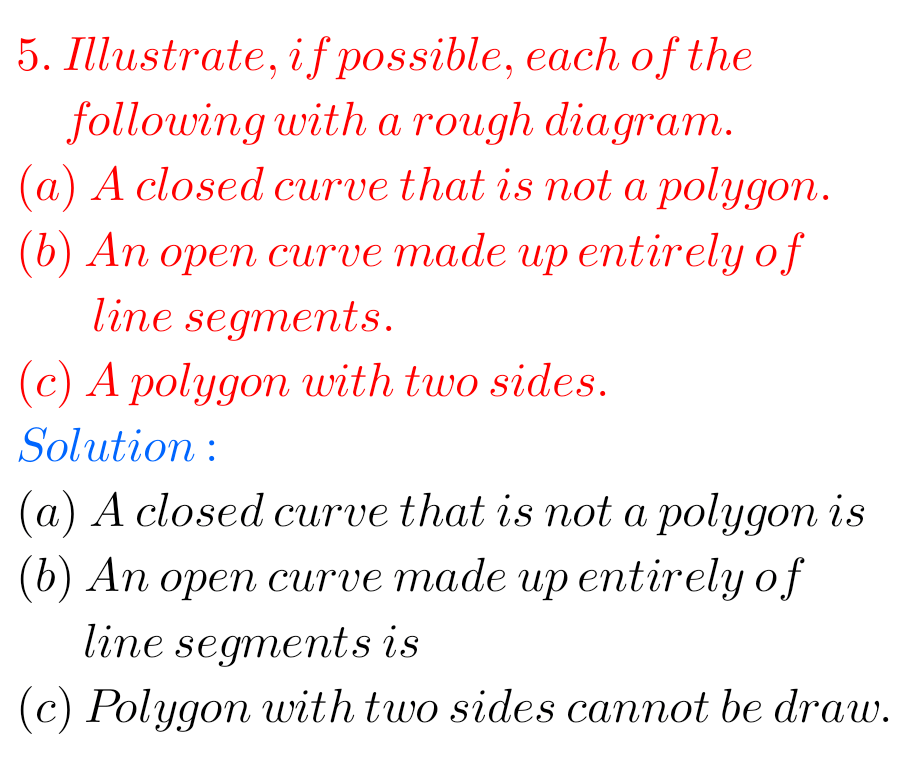## Maths Basic Geometrical Ideas Solutions exercise 4.2 class 6 Ncert

Basic Geometrical Ideas Solutions exercise 4.2 Class 6 Ncert Maths Ncert textbook Mathematics Class 6 Chapter 4 Basic Geometrical Ideas exercise 4.1 solutions are given. Study the lesson Basic Geometrical Ideas in the texbook. Observe the problems and solutions given in the text book. Observe the given below problems and solutions and try them in …## Ncert solutions for Maths Basic Geometrical Ideas Exercise 4.1 Class 6

Basic Geometrical Ideas Solutions exercise 4.1 Class 6 Ncert Maths Ncert textbook Mathematics Class 6 Chapter 4 Basic Geometrical Ideas exercise 4.1 solutions are given. Study the lesson Basic Geometrical Ideas in the texbook. Observe the problems and solutions given in the text book. Observe the given below problems and solutions and try them in …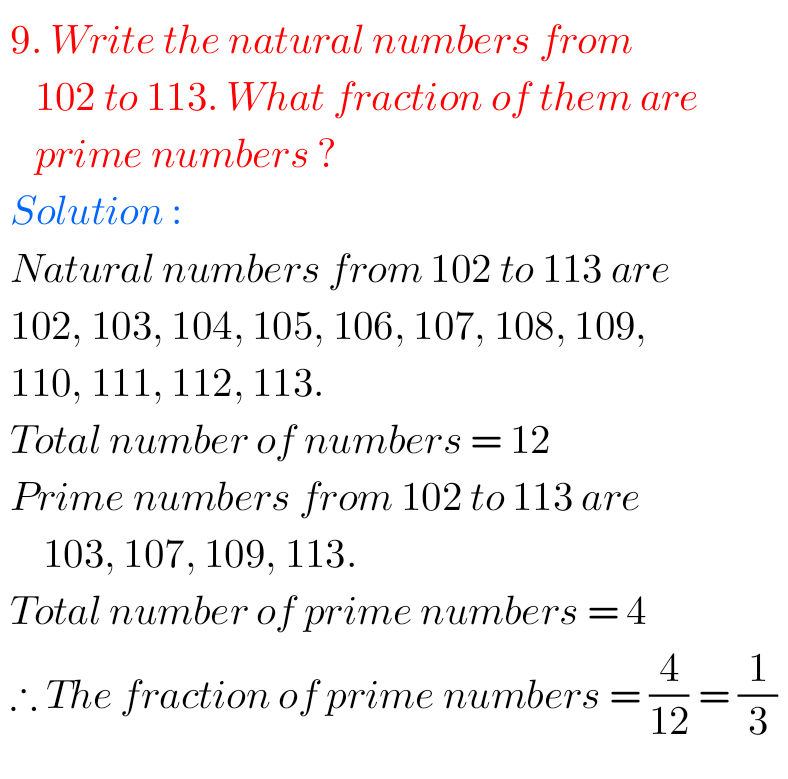## Fractions exercise 7.1 chapter 7 class 6 Maths solutions Ncert

Ncert solutions for Fractions exercise 7.1 class 6 Maths Ncert Ncert Maths Class 6 Chapter 7 exercise 7.1 problems with solutions are given below. Study the textbook lesson Fractions very well. Observe the example problems and solutions given in the textbook. Try them. Observe the given below solutions and try them in your own method  …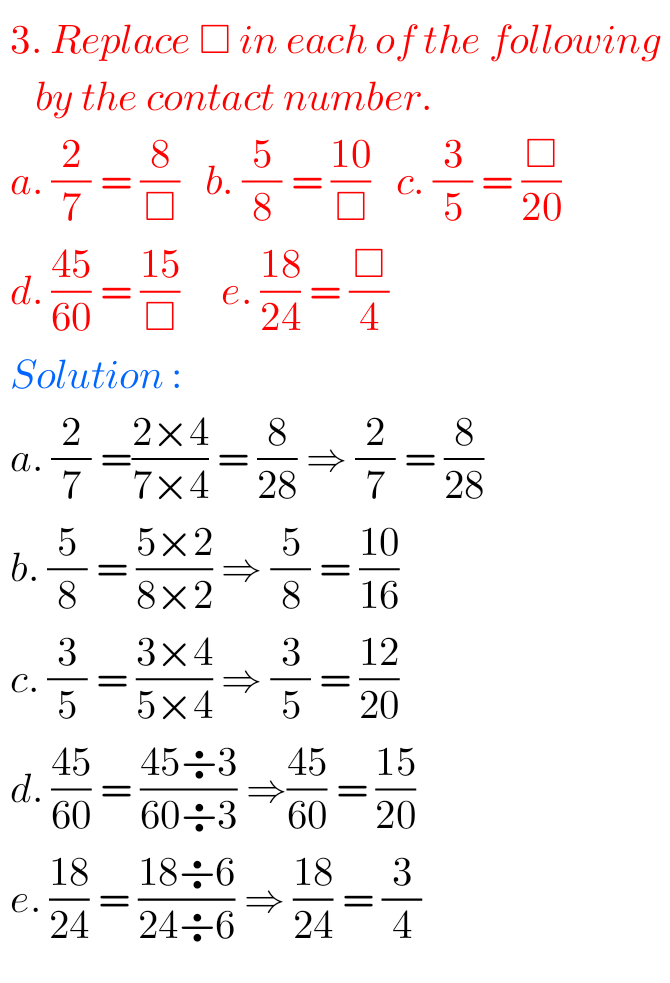## Maths Solutions for Ncert Fractions exercise 7.3

Ncert solutions for Fractions exercise 7.3 class 6 Maths Ncert Ncert Maths Class 6 Chapter 7 exercise 7.3 textbook problems with solutions are given below. Study the textbook lesson Fractions very well. Observe the example problems and solutions given in the textbook. Try them. Observe the given below solutions and try them in your own …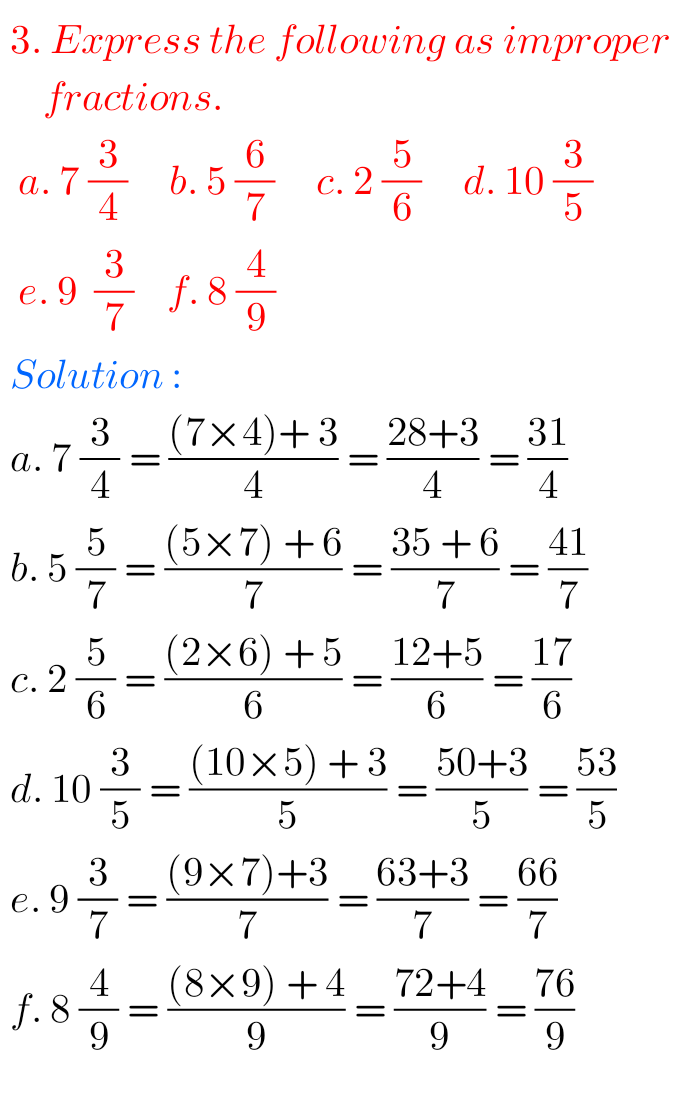## 6th class Maths Fractions exercise 7.2 solutions Ncert

Ncert solutions for Fractions exercise 7.2 class 6 Maths Ncert Ncert Maths Class 6 Chapter 7 exercise 7.2 problems with solutions are given below. Study the textbook lesson Fractions very well. Observe the example problems and solutions given in the textbook. Try them. Observe the given below solutions and try them in your own method  …